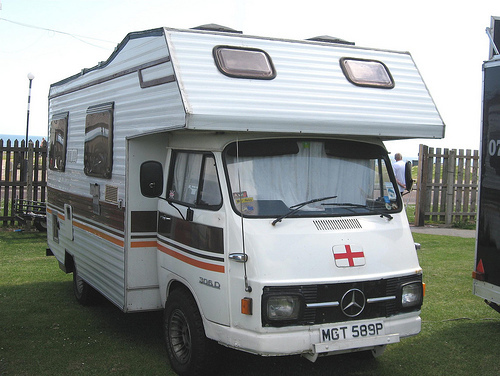# Got wrong results using Relay TensorRT Integration

Hello, all!

Based on `Relay TensorRT Integration` docs wrote by @trevor-m , I tried to deploy ResNet_v1_50 with Relay TensorRT Integration. The model is downloaded from tensorflow model zoo. And I used `relay.frontend.from_tensorflow` as the front end.

Unfortunately, I got the wrong results. In order to test the program, I selected several images in ImageNet validation dataset. No matter what input images I used, the inferenced classification ID was wrong which was always 111.

In the scripts uploaded, I also used both tensorflow and tensorrt to inference. And I can get correct results.

image classification ID relay tensorrt integration result tensorflow result tensorrt result
ILSVRC2012_val_00000012.JPEG 286 111 286 286
ILSVRC2012_val_00000014.JPEG 757 111 757 757

My development environments:
Ubuntu 16.04
TensorFlow 1.15.3
TensorRT 7.00.11

``````# doc: https://tvm.apache.org/docs/deploy/tensorrt.html
import cv2
import os
import numpy as np
import tensorflow as tf
import tvm
from tvm import relay
from tvm.relay.op.contrib.tensorrt import partition_for_tensorrt
from tensorflow.python.compiler.tensorrt import trt_convert as trt
from tvm_serving.frontend.tensorflow import graph_utils

image_name = "ILSVRC2012_val_00000014.JPEG"
output_node_names = ["resnet_v1_50/predictions/Reshape_1"]
layout = "NCHW"
inference_graph_def_path = "/root/code/optimize_object_detection_by_tvm/tvm_serving/examples/" \
"tensorrt/frozen_resnet_v1_50.pb"

_RESIZE_SIDE_MIN = 256
_RESIZE_SIDE_MAX = 512

def smallest_size_at_least(height, width, smallest_side):
height = float(height)
width = float(width)
smallest_side = float(smallest_side)
scale = 0.0
if height > width:
scale = smallest_side / width
else:
scale = smallest_side / height
new_height = int(np.rint(height * scale))
new_width = int(np.rint(width * scale))
return new_height, new_width

def aspect_preserving_resize(image, smallest_side):
shape = image.shape
height = shape
width = shape
new_height, new_width = smallest_size_at_least(height, width, smallest_side)
size = (new_width, new_height)
resized_image = cv2.resize(image, size, interpolation=cv2.INTER_CUBIC)
return resized_image

def central_crop(image, crop_height, crop_width):
image_height = image.shape
image_width = image.shape
offset_height = int((image_height - crop_height) / 2)
offset_width = int((image_width - crop_width) / 2)
cropped_image = image[offset_height:offset_height + crop_height, offset_width:offset_width + crop_width, :].copy()
return cropped_image

def get_input_from_cv2(image_path=os.path.join(".", image_name), image_size=224):
image = aspect_preserving_resize(image, 256)
image = central_crop(image, image_size, image_size)
return image

infer_graph_def = tf.GraphDef()
with open(graph_def_path, 'rb') as f:
return infer_graph_def

def from_graph_def_to_graph(graph_def):
g = tf.Graph()
with g.as_default():
tf.import_graph_def(graph_def, name='')
return g

def optimize_by_tvm_tensorrt():
x = get_input_from_cv2(os.path.join(".", image_name), 224)
x = np.expand_dims(x, 0)
input_name = "input"
dtype = "float32"
shape_dict = {input_name: x.shape}
mod, params = relay.frontend.from_tensorflow(graph_def, layout=layout,
shape=shape_dict, outputs=output_node_names)
mod, config = partition_for_tensorrt(mod, params)
target = "cuda"
with tvm.transform.PassContext(opt_level=3, config={'relay.ext.tensorrt.options': config}):
lib = relay.build(mod, target=target, params=params)
ctx = tvm.gpu(0)
gen_module = tvm.contrib.graph_runtime.GraphModule(lib['default'](ctx))
gen_module.run(data=tvm.nd.array(x.astype(dtype)))
output = gen_module.get_output(0).asnumpy()
classification = np.argmax(output)
print("classification: ", classification)

def run_graph_by_tensorflow():
graph = from_graph_def_to_graph(graph_def)
x = get_input_from_cv2(os.path.join(".", image_name), 224)
x = np.expand_dims(x, 0)
input_tensor = graph.get_tensor_by_name('input:0')
reshape = graph.get_tensor_by_name(output_node_names + ":0")
config = tf.ConfigProto()
config.gpu_options.allow_growth = False
config.gpu_options.per_process_gpu_memory_fraction = 0.5
with tf.Session(graph=graph, config=config) as sess:
output = sess.run(reshape, feed_dict={input_tensor: x})
classification = np.argmax(output)
print("classification: ", classification)

def optimize_by_trt():
x = get_input_from_cv2(os.path.join(".", image_name), 224)
x = np.expand_dims(x, 0)
converter = trt.TrtGraphConverter(input_graph_def=graph_def, nodes_blacklist=output_node_names)
trt_graph = converter.convert()
g = from_graph_def_to_graph(trt_graph)
input_tensor = g.get_tensor_by_name('input:0')
reshape = g.get_tensor_by_name(output_node_names + ":0")
with tf.Session(graph=g) as sess:
output = sess.run(reshape, feed_dict={input_tensor: x})
classification = np.argmax(output)
print("classification: ", classification)

if __name__ == '__main__':
run_graph_by_tensorflow()
optimize_by_trt()
optimize_by_tvm_tensorrt() # wrong result
``````Thanks for raising this issue. Could you please try changing layout to “NHWC” instead of “NCHW” when importing the TensorFlow model?

``````mod, params = relay.frontend.from_tensorflow(graph_def, layout="NHWC",
shape=shape_dict, outputs=output_node_names)
``````

The reason for this is that `from_tensorflow` does a very poor job of converting the layout and add many redundant operations. `partition_from_tensorrt` will already convert the layout to NCHW using the ConvertLayout pass in a very efficient way.

While neither of this should affect the accuracy, the extra redundant ops from `from_tensorflow` may be triggering a bug somewhere. I can look into that.

But after I changing layout to `"NHWC"`, the results are still wrong.

I figured out that maybe I misused `run` function. In my script, I call `run` like this:

``````gen_module.run(data=tvm.nd.array(x.astype(dtype)))
``````

But today I noticed that the correct usage is:

``````# x.astype(dtype) is a numpy.ndarray
gen_module.run(data=x.astype(dtype))
``````

or

``````# tvm.nd.array(x.astype(dtype) is a tvm.runtime.ndarray.NDArray
gen_module.set_input(input_name, tvm.nd.array(x.astype(dtype)))
gen_module.run()
``````